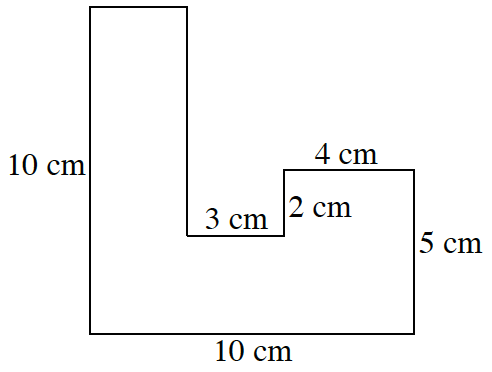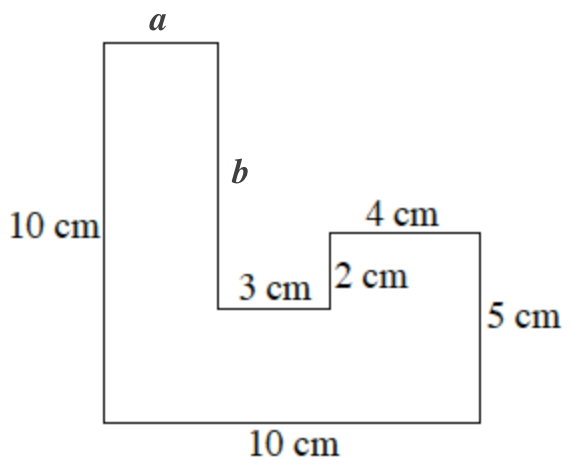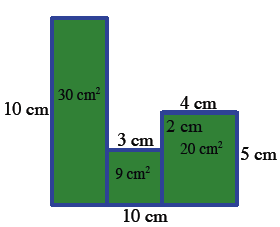### Home > ACC6 > Chapter 2 Unit 2 > Lesson CC1: 2.2.3 > Problem2-46

2-46.

Find the area and perimeter of the figure at right. All angles are right angles. Show all of your work.The perimeter of a figure is the sum of the lengths of all the sides.

To find the perimeter, find the lengths of the unknown sides (labeled $a$ and $b$ below). Find $a$ by noting that the sum of $a$, $3$ cm, and $4$ cm equals $10$ cm. Find $b$ in a similar manner.

Labels added to the unknown sides, top edge, a, right edge, b.The area of any square or rectangle is the length times the width. Divide the figure into squares and rectangles.  See the example below for one way to divide the figure.
Vertical segments, from top to bottom edges, aligned with the vertical sides, divide the interior area into 3 rectangles, Labels added to the 3 vertical rectangles, left, 30 square cm, middle, 9 square cm, right, 20 square cm.